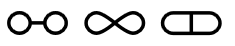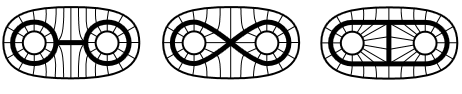Contents

Contents

Definition

A homotopy equivalence between topological spaces (or other objects in a category equipped with a notion of homotopy) $X$ and $Y$ is a morphism $f:X\to Y$ which has a homotopy inverse, hence such that there exists a morphism $g:Y\to X$ and homotopies $g \circ f \sim 1_X$ and $f \circ g \sim 1_Y$.

(Note the similarity with the strict notion of equivalence of categories.)

If there exists a homotopy equivalence between $X$ and $Y$ we say that $X$ and $Y$ are homotopy equivalent or that they have the same homotopy type. Being homotopy equivalent is evidently an equivalence relation.

For many purposes, one wants instead weak homotopy equivalences. Often when speaking of a homotopy equivalence or a homotopy type, one actually means the weak version. (This is especially likely on this wiki, due to the relationship to $\infty$-infinity-groupoids suggested by the homotopy hypothesis.)

The homotopy category of Top with respect to homotopy equivalences is Ho(Top)${}_{he}$.

Examples

Example

The following three graphs(i.e. the evident topological subspaces of the plane $\mathbb{R}^2$ that these pictures indicate) are not homeomorphic. But they are homotopy equivalent, in fact they are each homotopy equivalent to the disk with two points removed, by the homotopies indicated by the following pictures:graphics grabbed from Hatcher

More generally, in any (∞,1)-category $C$ other than Top, a homotopy equivalence in $C$ is just an equivalence in $C$.

Properties

Any homotopy equivalence is also a weak homotopy equivalence. Conversely, in the context of topological spaces, any weak homotopy equivalence between m-cofibrant spaces (spaces that are homotopy equivalent to CW complexes) is a homotopy equivalence. This is the Whitehead theorem.

The homotopy equivalences are the weak equivalences in the Strøm model structure on topological spaces. The homotopy category resulting from inverting all homotopy equivalences in Top is the same as that resulting from identifying homotopic maps.

Strong homotopy equivalences

Sometimes an apparently stronger form of homotopy equivalence is needed. These seem to have been first noticed by Richard Lashof in 1970 in work on triangulation and smoothing. The point is one of the interaction between the two homotopies, which are not made explicit in the definition above. Let us set this up slightly differently:

In a homotopy equivalence there are the two maps $f: X\to Y$ and $g: Y\to X$, then two homotopies

$H : X\times I \to X, H:g \circ f \sim 1_X$

and

$K: Y\times I \to Y, K : f \circ g \sim 1_Y.$

The whiskering actions of maps on homotopies (and more generally in any (∞,1)-category or category with a cylinder functor) gives two homotopies $f\circ g \circ f \sim f$, namely $f_*H = f\circ H : X\times I \to Y$ and $f^*K = K\circ (f\times I)$. Similarly, of course, there are two homotopies $g\circ f \circ g \sim g$, namely $g_*K$ and $g^*H$. In the usual definition of homotopy equivalence, there is no coherence required between these. That is handled precisely by the notion of strong homotopy equivalence. More precisely Lashof defined

Definition

A strong homotopy equivalence between spaces $X$ and $Y$ is a quadruple $(f,g,H,K)$, as above, such that $f_*H \sim f^*K$ and $g_*K\sim g^*H$.

Thus this imposes a minimal coherence condition on the data making up the homotopy equivalence. The definition clearly can be generalised to any reasonable setting with a notion of homotopy.

For example, in a 2-category where a homotopy is a natural isomorphism, and a homotopy between homotopies is just an equality, a homotopy equivalence is simply an equivalence, while a strong homotopy equivalence is the same as an adjoint equivalence.

The question naturally arises as to whether all homotopy equivalences are strong. Rainer Vogt(1972) proved

Vogt’s Lemma

If $f: X\to Y$ be a morphism that is a homotopy equivalence in $Top$, let $g: Y\to X$ be a homotopy inverse and $H:g \circ f \sim 1_X$ a homotopy. Then there is a homotopy $K: f \circ g \sim 1_Y$ such that $(f,g,H,K)$ is a strong homotopy equivalence.

Various versions of this are known in other settings, e.g. $SSet$-enriched categories. In a 2-category the corresponding fact is that any equivalence can be improved to an adjoint equivalence, by changing at most one of the 2-cell isomorphisms involved.

Note, though, that the coherence supplied by Vogt’s lemma is not required to continue to higher levels. There is no condition of compatibilty between the two ‘homotopies between the homotopies’. In some situations, at least, some higher compatibility is known to be derivable; for instance, any biequivalence in a 3-category can be improved to an adjoint biequivalence.

Tim: I do not know if there is a neat formulation of the full homotopy coherent version of this, nor exactly in what settings the analogous abstract versions of Vogt’s lemma go across

Mike Shulman: I think there’s a “full coherentification” version for quasicategories in HTT somewhere.

Vogt’s Lemma plays a key role in the proof of Vogt’s theorem (cf. homotopy coherent diagrams).